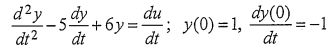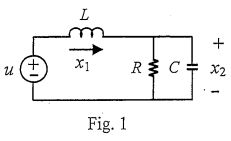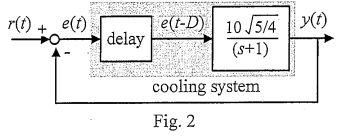lvy>試卷(2022/08/05)

# 102 年 - 102 國立中山大學_碩士班招生考試_電機系(乙組)：控制系統#110070

【非選題】
1.

PROBLEM 1(40%) Given a system described by the following differential equationwhere u is the control input and y is the output.

【題組】 (a) (10%) Find the transfer function of the system.

【非選題】
2.【題組】(b) (10%) Find the zero-input response y(t).

【非選題】
3.【題組】(c) (10%) Choose, among the following types of control, ones that are likely to stabilize the system (One or more answers may be correct. Answers without supporting explanation are not acceptable) : (i) P control (ii) I control (iii) D control (iv) PID control (v) PD control (vi) PI control

【非選題】
4.【題組】(d) (10%) Choose, among the following types of control, ones that are likely to regulate the system output to a constant level 0.5 with zero steady state error (One or more answers may be correct. Answers without supporting explanation are not acceptable): (1) P control (ii) I control (iii) D control (iv) PID control (v) PD control (vi) PI control

【非選題】
5.

PROBLEM 2 (30%) An LCR circuit is shown in Fig. 1, with its control input to be u, and output y = x2 .【題組】 (a) (10%) Derive a state-space representation of this LCR circuit.

【非選題】
6.【題組】(b) (10%) Suppose L =C=R=1 and x1(0)=x2(0)=1. What is the output y in steady state if u =sin(t)?

【非選題】
7.【題組】(c) (10%) Following 2(b), let the control be u(t)=-k1x1(t)-k2x2(t). Choose the gains k1 and k2 such that the closed loop poles are -2 and -3.

【非選題】
8.
PROBLEM 3 (20%)
Figure 2 shows a simple temperature control of a machine tool cooling system, where r is the setting temperature and y is the coolant temperature. There is a delay of D seconds present in the cooling

【題組】(a) (10%) Use Laplace transform to derive the transfer function from e(t) to e(t-D).

【非選題】
9.【題組】

(b) (10%) Estimate the maximum allowable delay for the system to maintain at least 20 dB of gain margin, by using the approximation, when |ω|<<1.

【非選題】
10.PROBLEM 4 (10%) After dozing off in the Monday morning class, your classmate asked you: "Integral control adds negative phase, thus decreasing the phase margin. Why would we ever want to use integral control?" Explain how you would answer him. system.